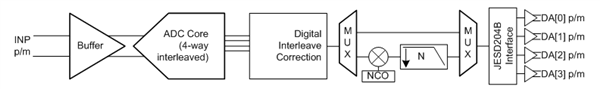# RF sampling: Learning more about latency

In this post, I’m going to discuss latency in an analog-to-digital converter (ADC). Latency is the time it takes for a signal to travel from point A to point B. In an ADC, latency is how long it takes from the time that an analog input is applied to the time that the digital output word becomes available.

Why is latency important? Regardless of the application, latency is a key specification as it determines the response time.  For example, data acquisition systems used in military applications are sensitive to the absolute latency, with lower being better. On the other hand, a known latency or deterministic latency is a key requirement for newer techniques like beam forming that is being adopted to improve sensitivity and selectivity in cellular communications systems.

Usually expressed in sampling clock cycles, latency naturally includes the time it takes for the ADC core to do the conversion. Conversion time is dependent on the ADC architecture; in a pipelined ADC, latency will depend on the number of internal stages in the pipeline, as opposed to successive-approximation register (SAR) converters that start transmitting the digital-output word within one or two clock periods.

In modern, high-speed, high-performance ADCs, the output of the ADC core (now in digital format) goes through much more signal processing, contributing significantly to latency. Figure 1 is a block diagram (signal chain only) for the ADC32RF45. Referring back to my definition of latency, in Figure 1, point A would be INP and point B would be DA, DA, DA and DA, (“p/m” denotes differential signaling). The propagation delay through different blocks (buffer, multiplexer), conversion time (in the ADC core), digital processing time (interleaving correction) and delay through the decimation filter (N) all contribute to the overall latency of the ADC.Figure 1: Block diagram of the ADC32RF45 (signal chain in brief)

Sampling rates overcoming the gigasample barrier have pushed output data rates even higher; for example, the ADC32RF45 operating at a maximum sample rate of 3GSPS puts out data at a rate of nearly 40Gbps which is clearly beyond what is possible with even a parallel low-voltage differential signaling (LVDS) interface. ADC32RF45 includes a JESD204B interface, which significantly reduces the number of interconnects required between the data converter and the receiver of the data, typically a field-programmable gate array (FPGA). With this interface, you need only four transmission lines between the data converter and the FPGA, and they need not be electrically matched. This significantly reduces pin count (and package size) in addition to making board design easier. As in most other cases, it does come with a penalty: additional delay adding to the latency. In the case of the JESD204B, the data is scrambled, 8b/10b encoded and finally serialized, all of which adds to the propagation delay – and all of which needs to be reversed at the receiver as well. This is a significant contributor to latency in the transmission of the data, even when lanes run at a full 12.5Gbps speed.

A digital down-conversion mixer followed by a decimation filter (N) is useful in cases where you don’t need the full Nyquist bandwidth or to reduce the lane rate.

ADC latency is therefore tightly related to the specific device and signal path followed. For the ADC32RF45, the lowest latency occurs when the digital down-conversion and decimation chain is bypassed (when the signal flows from one multiplexer directly to the other). This mode is called 14-bit 8224 bypass mode, and the latency in this case is expressed as Equation 1:

Latency8224_byp = 424 sampling clock cycles + 6ns                 (1)

Using the down-conversion and decimation chain adds to the latency, as shown in Table 1.

 Decimation option Additional latency (device clock cycles) Divide by 4 92 Divide by 6 322 Divide by 8 197 Divide by 9 339.5 Divide by 10 387 Divide by 12 473 Divide by 16 621 Divide by 18 740 Divide by 20 832 Divide by 24 1,019 Divide by 32 1,349